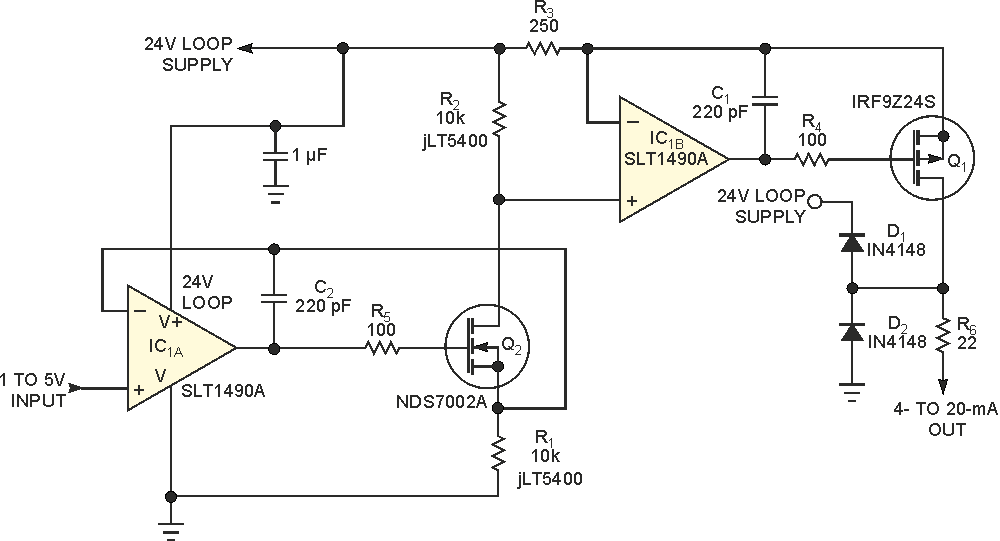# Convert 1 to 5V signal to 4- to 20-mA output

## Linear Technology LT5400

Thomas Mosteller, Linear Technology

EDN

Despite the long-predicted demise of the 4- to 20-mA current loop, this analog interface is still the most common method of connecting current-loop sources to a sensing circuit. This interface requires the conversion of a voltage signal – typically, 1 to 5 V – to a 4- to 20-mA output. Stringent accuracy requirements dictate the use of either expensive precision resistors or a trimming potentiometer to calibrate out the initial error of less precise devices to meet the design goals.

Neither technique is optimal in today’s surface-mounted, automatic-test-equipment-driven production environment. It’s difficult to get precise resistors in surface-mount packages, and trimming potentiometers require human intervention, a requirement that is incompatible with production goals.

The Linear Technology LT5400 quad matched resistor network helps to solve these issues in a simple circuit that requires no trim adjustments but achieves a total error of less than 0.2% (Figure 1). The circuit uses two amplifier stages to exploit the unique matching characteristics of the LT5400. The first stage applies a 1 to 5 V output – typically, from a DAC – to the noninverting input of op amp IC1A. This voltage sets the current through R1 to exactly VIN/R1 through FET Q2. The same current is pulled down through R2, so the voltage at the bottom of R2 is the 24 V loop supply minus the input voltage.Figure 1. Precision matched resistors provide accurate voltage-to-current conversion.

This portion of the circuit has three main error sources: the matching of R1 and R2, IC1A’s offset voltage, and Q2’s leakage. The exact values of R1 and R2 are not critical, but they must exactly match each other. The LT5400A grade achieves this goal with ±0.01% error. The LT1490A has less-than-700-μV offset voltage over 0 to 70 °C. This voltage contributes 0.07% error at an input voltage of 1 V. The NDS7002A has a leakage current of 10 nA, although it is usually much less. This leakage current represents an error of 0.001%.

The second stage holds the voltage on R3 equal to the voltage on R2 by pulling current through Q1. Because the voltage across R2 equals the input voltage, the current through Q1 is exactly the input voltage divided by R3. By using a precision 250 Ω current shunt for R3, the current accurately tracks the input voltage.

The error sources for the second stage are R3’s value, IC1B’s offset voltage, and Q1’s leakage current. Resistor R3 directly sets the output current, so its value is crucial to the precision of the circuit. This circuit takes advantage of the commonly used 250 Ω current-loop-completion shunt resistor. The Riedon SF-2 part in the figure has 0.1% initial accuracy and low temperature drift. As in the first stage, offset voltage contributes no more than 0.07% error. Q1 has less than 100-nA leakage, yielding a maximum error of 0.0025%.

Total output error is better than 0.2% without any trimming. Current-sensing resistor R3 is the dominant source of error. If you use a higher-quality device, such as the Vishay PLT series, you can achieve an accuracy of 0.1%. Current-loop outputs are subject to considerable stresses in use. Diodes D1 and D2 from the output to the 24 V loop supply and ground help protect Q1; R6 provides some isolation. You can achieve more isolation by increasing the value of R6, with the trade-off of some compliance voltage at the output. If the maximum output-voltage requirement is less than 10 V, you can increase R6’s value to 100 Ω, affording even more isolation from output stress. If your design requires increased protection, you can fit a transient-voltage suppressor to the output with some loss of accuracy due to leakage current.

This design uses only two of the four matched resistors in the LT5400 package. You can use the other two for other circuit functions, such as a precision inverter, or another 4- to 20-mA converter. Alternatively, you can place the other resistors in parallel with R1 and R2. This approach lowers the resistor’s statistical error contribution by the square root of two.

## Materials on the topic

EDN